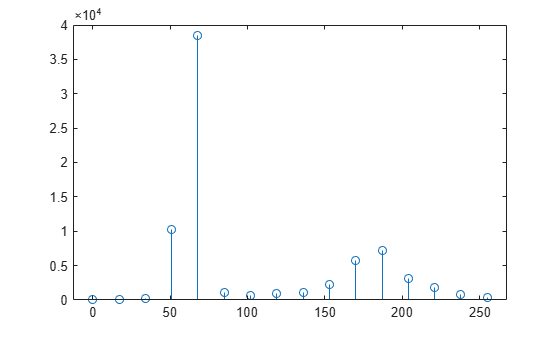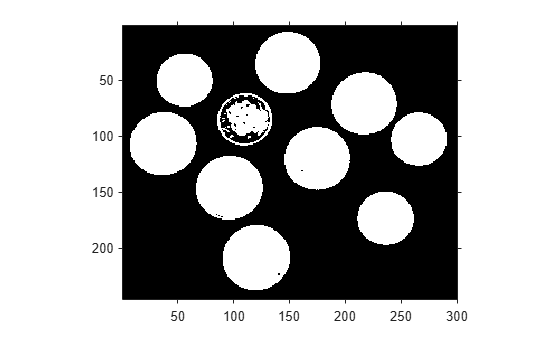# otsuthresh

Global histogram threshold using Otsu's method

## Syntax

``T = otsuthresh(counts)``
``````[T,EM] = otsuthresh(counts)``````

## Description

example

````T = otsuthresh(counts)` computes a global threshold `T` from histogram counts, `counts`, using Otsu's method . Otsu's method chooses a threshold that minimizes the intraclass variance of the thresholded black and white pixels. The global threshold `T` can be used with `imbinarize` to convert a grayscale image to a binary image.```
``````[T,EM] = otsuthresh(counts)``` returns the effectiveness metric, `EM`, which indicates the effectiveness of the thresholding.```

## Examples

collapse all

Read image into the workspace.

`I = imread('coins.png');`

Calculate a 16-bin histogram for the image.

```[counts,x] = imhist(I,16); stem(x,counts)```Compute a global threshold using the histogram counts.

`T = otsuthresh(counts);`

Create a binary image using the computed threshold and display the image.

```BW = imbinarize(I,T); figure imshow(BW)```## Input Arguments

collapse all

Histogram counts, specified as a vector of nonnegative numbers.

Data Types: `single` | `double` | `int8` | `int16` | `int32` | `int64` | `uint8` | `uint16` | `uint32` | `uint64`

## Output Arguments

collapse all

Global threshold, returned as a numeric scalar in the range [0, 1].

Data Types: `double`

Effectiveness metric of the threshold, returned as a numeric scalar in the range [0, 1]. The lower bound is attainable only by histogram counts with all data in a single non-zero bin. The upper bound is attainable only by histogram counts with two non-zero bins.

Data Types: `double`

 Otsu, N., "A Threshold Selection Method from Gray-Level Histograms." IEEE Transactions on Systems, Man, and Cybernetics. Vol. 9, No. 1, 1979, pp. 62–66.